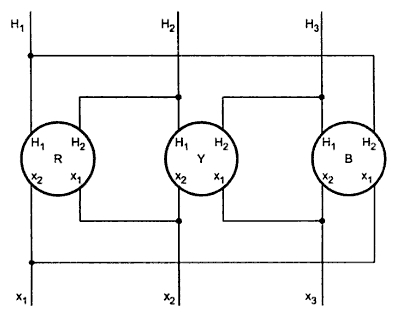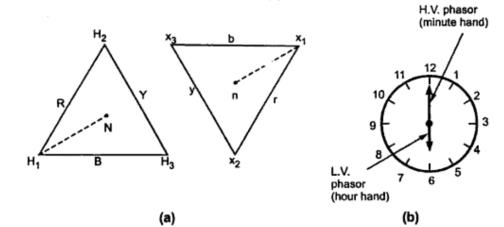### Construction of Vector Group Diagram

The polarity of each phase of a three phase transformer is obtained in similar way as that in case of single phase transformers. The voltage vector diagram is then constructed.
Consider a bank of three single phase transformers or a single unit of three phase transformer whose polarity is previously checked and is found to be additive. These transformers are connected in delta-delta fashion. This is shown in thew Fig. 1.Fig. 1  Three single phase transformers of additive polarity connected delta delta with 180o angular displacement

The corresponding vector diagram for this connection is shown in the Fig. 2(a). It is represented with clock method in the Fig. 2(b). Its symbol is Dd6.Fig. 2  (a) Voltage vecto9r diagram with clockwise phase rotation  (b) Clock method representation

The voltage vectors H1 - H2, H2 - H3 and H3 - H1 represents the induced voltages of the three phase transformers R, Y and B are displaced from each other by 120o. As the polarity is additive the low voltage vector of R i.e. nothing but vector x1 - x2 is opposing in direction to H1 - H2. Similar is the case with other two phases. The angular displacement between the high voltage and low voltage windings is the angle between the lines H1 - N and x1 - n where N and n are neutral points of voltage vector diagram. Here this angle is x1.
The low voltage vector diagram is constructed by drawing x1 - x2 opposite in direction to H1 - H2 . x2 - x3 in opposite direction to H2 - H3 while x3 - x1 in opposite direction to that of H3 - H1. This forms a closed delta. If the connections are not made properly then voltage diagram will not be closed to form a delta.

Related articles :
Sponsored links :